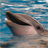# QlikView App Dev

Discussion Board for collaboration related to QlikView App Development.

Announcements
Customer & Partners, DEC. 9, 11 AM ET: Qlik Product & Strategy Roadmap Session: Data Analytics REGISTER NOW
cancel
Showing results for
Did you mean:Partner

## Site min and max based on region and product

Hi,

Here I'm attaching my excel sheet. In this sheet there is input data and also output what I'm expecting. And also I attached screenshot of input and output.

Could anyone help me on getting this requirement.

9 RepliesMVP

Create Pivot Table

Dimension:

Region

Valuelist('Min','Max')

Expression:

1) Product

Pick(Match(Valuelist('Min','Max'),'Min','Max'),

FirstSortedValue(Product, Den),

FirstSortedValue(distinct Product, - Den))

2) Value

Pick(Match(Valuelist('Min','Max'),'Min','Max'),

min(Den),

max(Den))Partner
Author

I can't see my result, instead it shows

 Region =Valuelist('Min','Max') Expr1 Expr2 Aus Min D 5 Aus Max C 2000MVP

Check it out:Script:

Table:

Region,

Product,

Num,

Den,

Year

FROM

tmpData.xlsx

(ooxml, embedded labels, table is Sheet1);

Dim:

Dim

1

2

];

Pivot Table:

Dimension

Region

=Pick(Dim, 'Max', 'Min')

Product

Expression:

=Pick(Dim,

If(Region = 'US',

Sum({<Key = {"=Den = Aggr(Max(TOTAL <Region, Year> Den), Region, Product, Year, Key)"}>} Den),

If(Region = 'UK',

Sum({<Key = {"=Num = Aggr(Max(TOTAL <Region, Year> Num), Region, Product, Year, Key)"}>} Num),

Sum({<Key = {"=Den - Num = Aggr(Max(TOTAL <Region, Year> Den - Num), Region, Product, Year, Key)"}>} Den - Num))),

If(Region = 'US',

Sum({<Key = {"=Den = Aggr(Min(TOTAL <Region, Year> Den), Region, Product, Year, Key)"}>} Den),

If(Region = 'UK',

Sum({<Key = {"=Num = Aggr(Min(TOTAL <Region, Year> Num), Region, Product, Year, Key)"}>} Num),

Sum({<Key = {"=Den - Num = Aggr(Min(TOTAL <Region, Year> Den - Num), Region, Product, Year, Key)"}>} Den - Num))))MVP

try this

Expression:

1) Product

Pick(Match(Valuelist('Min','Max'),'Min','Max'),

FirstSortedValue(Product, aggr(sum(Den),Region)),

FirstSortedValue(distinct Product, - aggr(sum(Den),Region))

2) Value

Pick(Match(Valuelist('Min','Max'),'Min','Max'),

FirstSortedValue(Den, aggr(sum(Den),Region)),

FirstSortedValue(distinct Den, - aggr(sum(Den),Region))MVP

Does not seem to be working KushPartner
Author

Sunny,

Nice technique but we are losing when we remove filters on year and filters on region or product. Here is the result with removing filter, based on my filters the chart needs to change. We can compare our output with below result by filtering and by removing filtering. So our needs to match with this rendering below chart.

 Region Product =Sum(Den) =Sum(Num) Aus A 220 110 B 440 330 C 2200 1100 D 55 44 UK A 124 120 B 20 18 C 130 126 D 14 12 US A 26 20 B 28 22 C 28 24 D 120 26MVP

I have not selected anything in Region or Product, so not sure I follow the logic for those two filters. With Year, what would you be seeing when nothing is selected? Max across the two years for a particular region or year or max between both years?MVP

I did not see any issue when we select Region, but with Product selection, things seemed wrong. Try this:

=Pick(Dim,

If(Region = 'US',

Sum({<Key = {"=Den = Aggr(Max({<Product>}TOTAL <Region, Year> Den), Region, Product, Year, Key)"}>} Den),

If(Region = 'UK',

Sum({<Key = {"=Num = Aggr(Max({<Product>}TOTAL <Region, Year> Num), Region, Product, Year, Key)"}>} Num),

Sum({<Key = {"=Den - Num = Aggr(Max({<Product>}TOTAL <Region, Year> Den - Num), Region, Product, Year, Key)"}>} Den - Num))),

If(Region = 'US',

Sum({<Key = {"=Den = Aggr(Min({<Product>}TOTAL <Region, Year> Den), Region, Product, Year, Key)"}>} Den),

If(Region = 'UK',

Sum({<Key = {"=Num = Aggr(Min({<Product>}TOTAL <Region, Year> Num), Region, Product, Year, Key)"}>} Num),

Sum({<Key = {"=Den - Num = Aggr(Min({<Product>}TOTAL <Region, Year> Den - Num), Region, Product, Year, Key)"}>} Den - Num))))Partner
Author

In the above result I removed all filters in app.

Eg:

for Aus

Max is C->2200 and Min is D->55

for UK

Max is C->130 and Min is D->14

for US

Max is D -> 120 and Min is A->26

When add filter the table will render. Based on that result our output needs to matchCommunity Browser